In this blog post, I compare the two ways in which MaxDiff data is treated in analyses such as latent class analysis and Hierarchical Bayes. The first, tricked logit, is a quick and dirty approach: it is fast, simple and convenient, but it does not correctly model the probability of choices in a MaxDiff questionnaire. The second, ranked-ordered logit with ties, is the righteous approach: it may be slower and more complicated, but it provides a correct probabilistic treatment for MaxDiff. To help decide which to use, I will compare empirical results from a MaxDiff data set.

## Tricked logit

Multinomial logit is used to model data where respondents have selected one out of multiple alternatives. The logit probability of selecting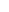given the utilities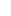is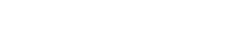where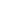denotes the set of alternatives.

In MaxDiff, respondents select two alternatives instead: their favourite (best) and least favourite (worst). Tricked logit models MaxDiff data by treating the best and worst selections as independent. The probability of the best selection is given by (1) whereas the probability for the worst selection is obtained by negating the utilities in (1):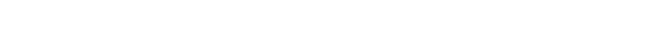This implies that the alternative that is most likely to be chosen as the best, is least likely to be chosen as the worst, and vice versa. However, this assumption of independence between the best and worst selections is unreasonable, as it does not rule out impossible scenarios where the same alternative is selected as both the best and worst.

So, why do people use tricked logit? It can be conducted by "tricking" existing software for multinomial logit into modeling the best and worst selections. This is done by duplicating the design matrix for the worst selections, except with the indicator value 1 replaced with -1. This approach is equivalent to (2). Another benefit is speed: with tricked logit the number of cases is only double that of multinomial logit, whereas the more correct model, rank-ordered logit with ties, is much more computationally intensive, as I will show below.

## Rank-ordered logit with ties

Rank-ordered logit with ties is applied to situations where respondents are asked to rank alternatives from best to worst, with the possibility of ties. MaxDiff data can be analysed using rank-ordered logit with ties since selecting the best and worst alternatives is the same as ranking the best alternative first, the worst alternative last, and the other alternatives tied in second place. To compute the probability of selecting a particular pair of best and worst alternatives, the probabilities of every possible way in which the respondent could have ranked the alternatives that would have led to the observed best and worst alternatives are summed together. It can be shown (I shall not attempt to do so here) that the probability is given bywhere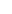is the set of combinations of alternatives other than the best and worst and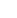denotes the number of alternatives in. As the number of combinations inis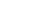, the cost of computing the probability increases exponentially with the number of alternatives.

## Prediction accuracies for Latent Class Analysis

I have compared both methods using the out-of-sample prediction accuracies for individual respondents with the technology companies dataset used in previous blog posts on MaxDiff. One of the simplest models to test on is latent class analysis. The line chart below plots prediction accuracies for both methods for a latent class analysis with five classes over random seeds 1 to 100. The models were fitted using four out of six of the questions given to each respondent and prediction accuracies were obtained from the remaining two questions. The seeds were used to generate the random selection of questions to be used in-sample and out-of-sample, so that different sets of questions are used with each seed. The line chart suggests that the two methods have a similar level of prediction accuracy. The mean difference between them is 0.14%, while the standard error of this mean is 0.16%. As the standard error of the mean is larger than the mean I consider their difference to be insignificant from zero and therefore they are tied in this comparison.

## Prediction accuracies for Hierarchical Bayes

Hierarchical Bayes is a much more flexible model compared to latent class analysis and it provides superior out-of-sample predictive performance. This can be seen by comparing the previous chart with the line chart below for Hierarchical Bayes: the prediction accuracy is around 10 percentage points higher. The correlation between the accuracies from both methods is higher too at 92% compared to 60%. Again, the two methods have a similar level of prediction accuracy. The mean difference between them is 0.06%, while the standard error of this mean is also 0.06%, which implies their difference is insignificant from zero.

## Estimated parameters

The results so far have indicated that the two methods give similar results. The radar charts below show the mean (absolute value) and standard deviation parameter values for each alternative, averaged over 20 random seeds. Tricked logit has slightly larger mean parameters and slightly larger standard deviations. I think this is due to the different way in which the parameters are converted to probabilities. For the same set of parameters, I have found that rank-ordered logit tends to yield higher probability. So, to compensate for this, their parameters tend to be closer to zero. I wouldn't read too much into this difference as it probably does not impact results.

## Summary

While rank-ordered logit is better supported by theory than tricked logit, I have found that the two perform the same when it comes to predicting individual responses for MaxDiff. As tricked logit is less computationally intensive, I would recommend using it when analysing MaxDiff data. Note that MaxDiff is only a trivial problem for rank-ordered logit, which is able to be applied in more general situations where respondents are asked to rank alternatives with the possibility of ties, and shortcut solutions such as tricked logit do not exist. To run tricked logit and rank-ordered logit in Displayr on the data used in this blog post, or on your own data set, click here.